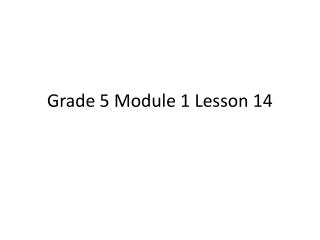DownloadDownload PresentationGrade 5 Module 1 Lesson 14

Grade 5 Module 1 Lesson 14

Download PresentationGrade 5 Module 1 Lesson 14

- - - - - - - - - - - - - - - - - - - - - - - - - - - E N D - - - - - - - - - - - - - - - - - - - - - - - - - - -
Presentation Transcript

1. Grade 5 Module 1 Lesson 14

2. Multiply and Divide by Exponents Write 65 tenths as a decimal on your place value chart.

3. Multiply and Divide by Exponents Write 65 tenths as a decimal on your place value chart. Say the decimal

4. Multiply and Divide by Exponents Write 65 tenths as a decimal on your place value chart. Say the decimal 6.5

5. Multiply and Divide by Exponents Write 65 tenths as a decimal on your place value chart. Say the decimal 6.5 Multiply by 102

6. Multiply and Divide by Exponents Write 65 tenths as a decimal on your place value chart. Say the decimal 6.5 Multiply by 102 Move digits 2 places to the left (650)

7. Multiply and Divide by Exponents Write 7 tenths as a decimal on your place value chart. Say the decimal

8. Multiply and Divide by Exponents Write 7 tenths as a decimal on your place value chart. Say the decimal 0.7 Multiply by 102

9. Multiply and Divide by Exponents Write 7 tenths as a decimal on your place value chart. Say the decimal 0.7 Multiply by 102 Move digits 2 places to the left (70)

10. Round to Different Place Values Say this number: 6.385

11. Round to Different Place Values Say this number: 6.385 6 and 385 thousandths On your boards, round the number to the nearest tenth.

12. Round to Different Place Values Say this number: 6.385 6 and 385 thousandths On your boards, round the number to the nearest tenth. 6.385 ≈ 6.4

13. Round to Different Place Values 6.385 6 and 385 thousandths On your boards, round the number to the nearest hundredths.

14. Round to Different Place Values 6.385 6 and 385 thousandths On your boards, round the number to the nearest hundredths. 6.385 ≈ 6.39

15. Round to Different Place Values Say this number: 37.645

16. Round to Different Place Values Say this number: 37.645 37 and 645 thousandths On your boards, round the number to the nearest tenth.

17. Round to Different Place Values Say this number: 37.645 37 and 645 thousandths On your boards, round the number to the nearest tenth. 37.645 ≈ 37.6

18. Find the Quotient 14 ÷ 2 = ___ (Write the division sentence)

19. Find the Quotient 14 ÷ 2 = ___ (Write the division sentence) 14 ÷ 2 = 7 Say the division sentence in unit form.

20. Find the Quotient 14 ÷ 2 = ___ (Write the division sentence) 14 ÷ 2 = 7 Say the division sentence in unit form. 14 ones ÷ 2 = 7 ones

21. Find the Quotient 1.4 ÷ 2 = ___ (Write the division sentence)

22. Find the Quotient 1.4 ÷ 2 = ___ (Write the division sentence) 1.4 ÷ 2 = 0.7 Say the division sentence in unit form.

23. Find the Quotient 1.4 ÷ 2 = ___ (Write the division sentence) 1.4 ÷ 2 = 0.7 Say the division sentence in unit form. 14 tenths ÷ 2 = 7 tenths

24. Application A bag of potato chips contains 0.96 grams of sodium. If the bag is split into 8 equal servings, how many grams of sodium will each serving contain?

25. Concept Development 6.72 ÷ 3 = ___ (Show 6.72 on your place value chart using the number disks)

26. Concept Development 5.16 ÷ 4 = ___ (Show 5.16 on your place value chart using the number disks)

27. Concept Development 6.72 ÷ 4 = ___ (Show 6.72 on your place value chart using the number disks)

28. Concept Development 20.08 ÷ 8 = ___ (Show 20.08 on your place value chart using the number disks)

29. Concept Development 6.372 ÷ 6 = ___ (Work independently using the standard algorithm to solve.)

30. Problem Set For 10 minutes, do your personal best to complete the Problem Set.

31. Student Debrief Lesson Objective: Divide decimals with a remainder using place value understanding and relate to a written method. How are dividing decimals and dividing whole numbers similar? How are they different?